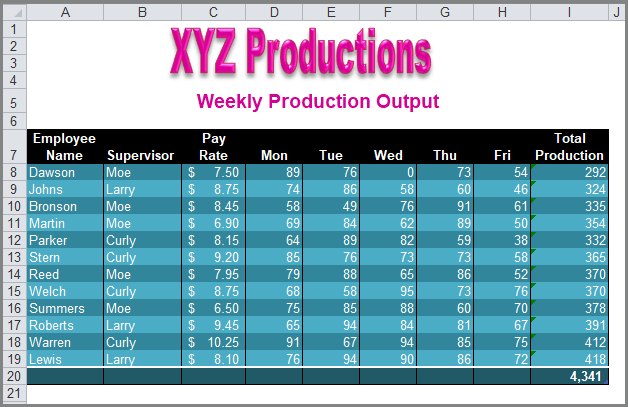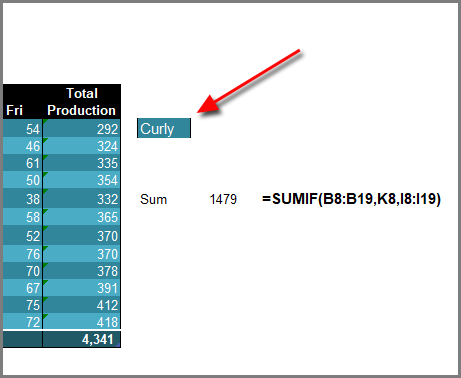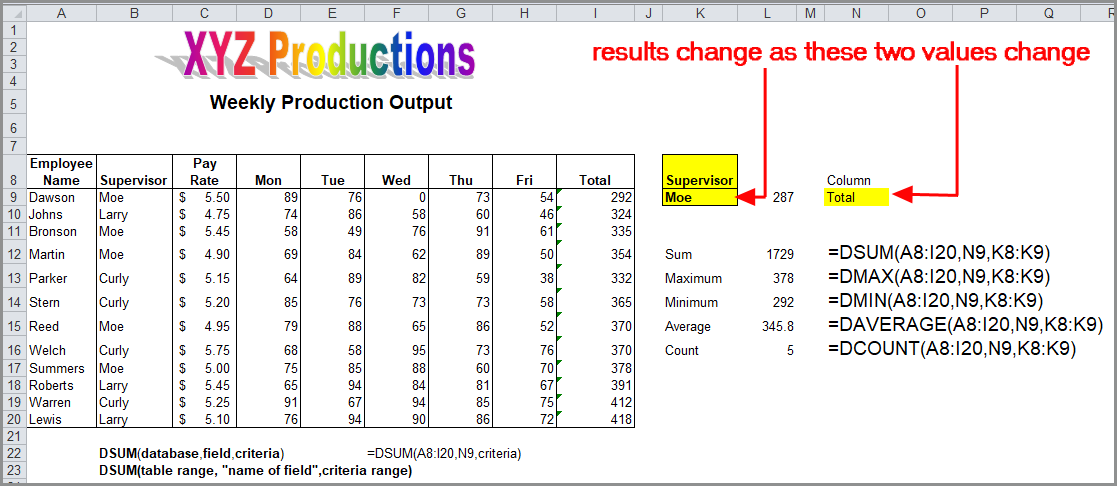Select Page

Share

If you’re an Excel user, you’ve probably used the SUM function. It’s fairly simple. The following formula returns the sum of all values in the range of cells in Column A from rows 4 through 1799:

=Sum(A4:A1799)

Sometimes you need to sort your data before you can get the totals you want.  But let’s take a look at some alternatives that might make your work day easier.

### SUMIF()

Maybe you’ve seen the =SUMIF function and wondered if it could help you.  Here’s how it works.  SUMIF allows you to calculate a conditional sum, including values in a range that meet one or more conditions.  For example, the following example shows production values for a company that runs three shifts.There is a Sum function in cell I20 that calculates Total Production.  But what if you wanted a total only for first shift?  This is where SUMIF would help.  The syntax for SUMIF is as follows:

SUMIF( range, criteria, [sum_range] )

Range: The range of cells that you want to apply the criteria against.
Criteria: The criteria used to determine which cells to add.
[Sum_range]: Optional. It is the range of cells to sum together. If this parameter is omitted, it uses range as the sum_range.

In the following illustration, you can see the SUMIF formula in cell L8.  It uses range B8:B19 as the range to search in for the criteria (the Supervisor column), and cell K8 as the criteria, which currently contains the value “Moe.”  The sum-range is I8:I19, which contains the values that we want totaled (Production).To change the criteria, enter the name of  a different shift supervisor in cell K8.  The SUMIF formula will recalculate the total.In addition to SUMIF(), you can also use AVERAGEIF(), which will calculate the average and COUNTIF(), to count the entries. The syntax for AVERAGEIF is the same as for SUMIF:

AVERAGEIF (range, criteria, [average_range])

The syntax if COUNTIF is slightly different:

COUNTIF (range, criteria)### DSUM() and other Database Statistics

Excel also contains a set of database statistical functions.  DSUM is similar to SUMIF in that it only adds up items that fulfill a certain criteria.  For example, in the following illustration you can use the database stastical functions as follows:

• DSUM would total all hours worked for just employees who work for one of the 3 managers.
• DMAX would find the highest of the values for the criteria specified.
• DMIN would find the lowest of the values for the criteria specified.
• DAVERAGE would average them.
• DCOUNT would count them.All the database statistical functions (DSum, DAverage, DMax, DMin, DCount) have the following syntax:

DXXX (database, field, criteria)

In the example above, when you enter a different manager name in cell K9 the formulas in column L will all return different values based on the revised criteria. Similarly, when you enter a different column name in cell N9 (like Mon, Tue, etc.) the formulas in column L will all return different values based on the different field name.

So to sum up (no pun intended), without using conditional statistics you would need to sort by manager and then summarize the employees.  These functions make that unnecessary.

# 1 Comment

1.Thank you so much! I’m glad it was helpful. You are more than welcome to share the post.